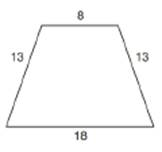Chapter 8.5, Problem 27E### Elementary Geometry for College St...

6th Edition
Daniel C. Alexander + 1 other
ISBN: 9781285195698

#### Solutions

Chapter
Section### Elementary Geometry for College St...

6th Edition
Daniel C. Alexander + 1 other
ISBN: 9781285195698
Textbook Problem
4 views

# A circle can be inscribed in the trapezoid shown. Find the area of that circle.To determine

To find:

Area of circle inscribed in a trapezoid.

Explanation

Formula:

If r is the radius of circle,

Acircle=πr2.

Calculation:

Consider the following diagram in which ABCD is a trapezoid with an inscribed circle.

Two perpendiculars AM and DN have been drawn from vertices A and D of the trapezoid.

Consider AMB and DNC.

In these two triangles, AB=DC=13

AMB=DNC=90°.

We know that the distance between parallel lines is same.

Hence, AM=DN.

From the above observations, it is clear that AMBDNC by RHS (right-angle hypotenuse side) test of congruency.

We know that, if two triangles are congruent then their corresponding sides are also equal.

Hence, BM=NC.

In quadrilateral AMND, AM=DN and AMN=DNM=90°,

Hence, AMND is a rectangle.

Opposite sides of a rectangle are equal. Therefore, AD=MN=8

Points M and N lie on BC. Hence, BC can be written as the sum of three segments.

BC=BM+MN+NC

18=BM+8+NC

Thus, BM+NC=18-8=10

Since, BM=NC

### Still sussing out bartleby?

Check out a sample textbook solution.

See a sample solution

#### The Solution to Your Study Problems

Bartleby provides explanations to thousands of textbook problems written by our experts, many with advanced degrees!

Get Started